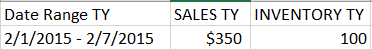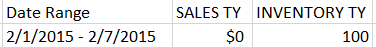# QlikView App Development

Discussion Board for collaboration related to QlikView App Development.

Announcements
Make your voice heard! Participate in the 2020 Wisdom of Crowds® Survey. BEGIN SURVEY
HighlightedNot applicable

## Set Analysis Question

We have a Sales and Inventory QVW document in which we have a tab where you can select a year,month and week number from a list box. Also we have a Multi Box for selecting information related to a Product like - SKU number, Product Class etc. We have another Multi Box for information related to Store like - Store Number, Store Name etc.

There is a Pivot Chart which reflects the change in data according to the selections made in these list boxes and Multi Boxes. The Pivot Chart has the following columns - Date Range (Week), Sales for current year and Inventory for current year.

Example:

I want to be able to pick a Product SKU and a week number that will c

When selected a SKU - '500'What I want to achieve:

When selected SKU - '500' and then pick a store number - '309'. The store 309 had no transactions but we still want to see the Total Inventory although the Sales would show 0.What's not Working:

Because there were no transaction in that store, all the selections get dis-selected  and the Inventory changes to 0 too. And we don't want this to change to 0. It should still show 100.How can I achieve this? So if there were no transactions in that particular store, the sales should change to 0 but the Inventory should not. It should still show the Total Inventory irrespective of the Store selection.

NOTE: INVENTORY TY is a metric.

I think this can be achieved using Set Analysis but I'm not sure how to implement it. Thanks for your help!!

9 Replies
HighlightedNot applicable

## Re: Set Analysis Question

Hi Vidit

You are correct, this can be achieved with set analysis. The will want update your Inventory measure calculation to use something similar to the following expression, updating the Store ID to your field name as appropriate.

=SUM( {\$<[STORE ID]=>} [INVENTORY TY])

The part highlighted in bold is the set analysis that you will want to add to your aggregation calculation so that store selections are disregarded for the measure.

Reply or update post if this helps or not.

Kind regards

HighlightedNot applicable

## Re: Set Analysis Question

Hi Steven,

Thanks for a quick reply! I tried to add the set analysis part you gave above to my metric calculation (which is also a set analysis function) but did not seem to work. Am I doing anything wrong?

So Store_Key is the primary key of my Store table.

Original Metric Calculation:

sum({\$<inv_retail_year={\$(=max(Total retail_year))}, inv_retail_week_num={\$(=min(Total retail_week_num))}>} FIELD_INV_CURRENT) + sum({\$<inv_retail_year={\$(=max(Total retail_year))}, inv_retail_week_num={\$(=min(Total retail_week_num))}>} WAREHOUSE_INV) + sum({\$<inv_retail_year={\$(=max(Total retail_year))}, inv_retail_week_num={\$(=min(Total retail_week_num))}>} FIELD_INTRANSIT) + sum({\$<inv_retail_year={\$(=max(Total retail_year))}, inv_retail_week_num={\$(=min(Total retail_week_num))}>} WH_INTRANSIT)

sum({\$<[STORE_KEY]=>}{\$<inv_retail_year={\$(=max(Total retail_year))}, inv_retail_week_num={\$(=min(Total retail_week_num))}>} FIELD_INV_CURRENT) + sum({\$<[STORE_KEY]=>}{\$<inv_retail_year={\$(=max(Total retail_year))}, inv_retail_week_num={\$(=min(Total retail_week_num))}>} WAREHOUSE_INV) + sum({\$<[STORE_KEY]=>}{\$<inv_retail_year={\$(=max(Total retail_year))}, inv_retail_week_num={\$(=min(Total retail_week_num))}>} FIELD_INTRANSIT) + sum({\$<[STORE_KEY]=>}{\$<inv_retail_year={\$(=max(Total retail_year))}, inv_retail_week_num={\$(=min(Total retail_week_num))}>} WH_INTRANSIT)

Thanks

Vidit

HighlightedMVP

## Re: Set Analysis Question

Hi Vidit, syntax should be:

Sum({<Set analysis>} Field)

You're using this syntax, wich is wrong:

Sum({<Set analysis>}{<Set analysis>} Field)

So, it should be:

sum({\$<[STORE_KEY]=, inv_retail_year={\$(=max(Total retail_year))}, inv_retail_week_num={\$(=min(Total retail_week_num))}>} FIELD_INV_CURRENT)

HighlightedNot applicable

## Re: Set Analysis Question

Thanks Ruben! But it did not work.HighlightedMVP

## Re: Set Analysis Question

Hi Vidit, you changed the rest of the expression? Mine was only an example in the first Sum, but all the other must be changed in the same way.

Also, this wouldn't solve your problem with de-selecting when you select a store without sales, it should need a more complex solution, generating missing data or finding a way that keeps relation between products and stores even if they have no sales of that particular product.

Check this link, maybe it helps you:

Generating Missing Data In QlikView

Highlighted
Valued Contributor

## Re: Set Analysis Question

Hi, Vidit!

HighlightedNot applicable

## Re: Set Analysis Question

Thanks Ruben!

HighlightedNot applicable

## Re: Set Analysis Question

Hi Capren! I'm not sure how to attach a qvw to this post. But this is the calculation I was using:

sum({\$<[STORE_KEY]=>}{\$<inv_retail_year={\$(=max(Total retail_year))}, inv_retail_week_num={\$(=min(Total retail_week_num))}>} FIELD_INV_CURRENT) + sum({\$<[STORE_KEY]=>}{\$<inv_retail_year={\$(=max(Total retail_year))}, inv_retail_week_num={\$(=min(Total retail_week_num))}>} WAREHOUSE_INV) + sum({\$<[STORE_KEY]=>}{\$<inv_retail_year={\$(=max(Total retail_year))}, inv_retail_week_num={\$(=min(Total retail_week_num))}>} FIELD_INTRANSIT) + sum({\$<[STORE_KEY]=>}{\$<inv_retail_year={\$(=max(Total retail_year))}, inv_retail_week_num={\$(=min(Total retail_week_num))}>} WH_INTRANSIT)

HighlightedNot applicable

## Re: Set Analysis Question

Ruben, yes I did change the whole expression accordingly but it did not work either.